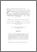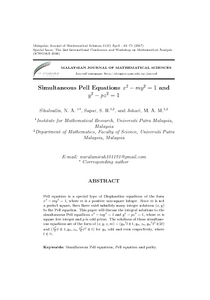# Simultaneous Pell equations x2 - my2 = 1 and y2 - pz2 = 1

## Citation

Sihabudin, Nurul Amirah and Sapar, Siti Hasana and Mohamad Johari, Mohamad Aidil (2017) Simultaneous Pell equations x2 - my2 = 1 and y2 - pz2 = 1. Malaysian Journal of Mathematical Sciences, 11 (spec. Apr.). pp. 61-71. ISSN 1823-8343; ESSN: 2289-750X

## Abstract

Pell equation is a special type of Diophantine equations of the form x2 − my2 = 1, where m is a positive non-square integer. Since m is not a perfect square, then there exist infinitely many integer solutions (x, y) to the Pell equation. This paper will discuss the integral solutions to the simultaneous Pell equations x2 − my2 = 1 and y2 − pz2 = 1, where m is square free integer and p is odd prime. The solutions of these simultaneous equations are of the form of (x, y, z, m) = (yn2t±1, yn, zn, yn2t2±2t) and (y2n/2 t ±1, yn, zn, y2n/4 t2) for yn odd and even respectively, where t ∈ N.Preview
PDF
6. Amiranhasana.pdfView Item